#### A one-dimensional variety

The most interesting and complicated situations occur when there is a continuum of solutions. For example, if one of the constraints is removed, then a one-dimensional set of solutions can be obtained. Suppose only one variable is constrained for the example in Figure 4.22. Intuitively, this should yield a one-dimensional variety. Set thecoordinate to 0, which yields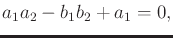(4.65)

and allow any possible value for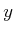. As shown in Figure 4.23a, the point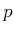must follow the-axis. (This is equivalent to a three-bar linkage that can be constructed by making a third joint that is prismatic and forced to stay along the-axis.) Figure 4.23b shows the resulting variety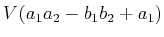but plotted in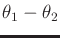coordinates to reduce the dimension from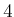to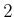for visualization purposes. To correctly interpret the figures in Figure 4.23, recall that the topology is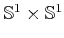, which means that the top and bottom are identified, and also the sides are identified. The center of Figure 4.23b, which corresponds to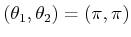, prevents the variety from being a manifold. The resulting space is actually homeomorphic to two circles that touch at a point. Thus, even with such a simple example, the nice manifold structure may disappear. Observe that at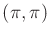the links are completely overlapped, and the pointof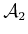is placed at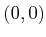in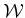. The horizontal line in Figure 4.23b corresponds to keeping the two links overlapping and swinging them around together by varying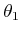. The diagonal lines correspond to moving along configurations such as the one shown in Figure 4.23a. Note that the links and the-axis always form an isosceles triangle, which can be used to show that the solution set is any pair of angles,,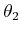for which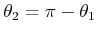. This is the reason why the diagonal curves in Figure 4.23b are linear. Figures 4.23c and 4.23d show the varieties for the constraints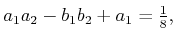(4.66)

and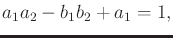(4.67)

respectively. In these cases, the point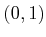inmust follow the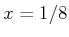and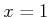axes, respectively. The varieties are manifolds, which are homeomorphic to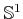. The sequence from Figure 4.23b to 4.23d can be imagined as part of an animation in which the variety shrinks into a small circle. Eventually, it shrinks to a point for the case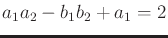, because the only solution is when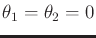. Beyond this, the variety is the empty set because there are no solutions. Thus, by allowing one constraint to vary, four different topologies are obtained: 1) two circles joined at a point, 2) a circle, 3) a point, and 4) the empty set.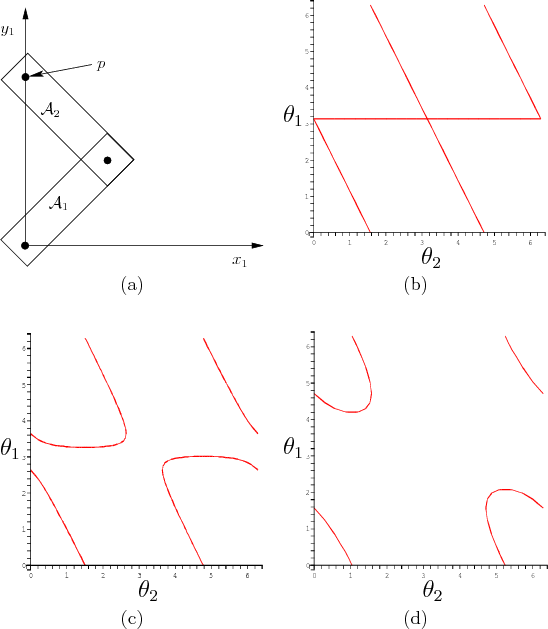Steven M LaValle 2020-08-14